## Sample Test Problems

1. A beam of 100 eV (kinetic energy) electrons is incident upon a potential step of heighteV. Calculate the probability to be transmitted. Get a numerical answer.
2. * Find the energy eigenstates (and energy eigenvalues) of a particle of massbound in the 1D potential. Assumeis a positive real number. (Don't assume thathas the units of energy.) You need not normalize the state.3. * A beam of particles of wave-number(this means) is incident upon a one dimensional potential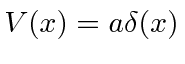. Calculate the probability to be transmitted. Graph it as a function of.
To the left of the origin the solution is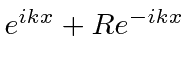. To the right of the origin the solution is. Continuity ofat the origin implies. The discontinuity in the first derivative isTransmission probability starts at zero forthen approaches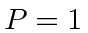asymptotically for.
4. * A beam of particles of energycoming from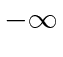is incident upon a delta function potential in one dimension. That is.
a)
Find the solution to the Schrödinger equation for this problem.
b)
Determine the coefficients needed to satisfy the boundary conditions.
c)
Calculate the probability for a particle in the beam to be reflected by the potential and the probability to be transmitted.
5. * The Schrödinger equation for the one dimensional harmonic ocillator is reduced to the following equation for the polynomial:a)
Assume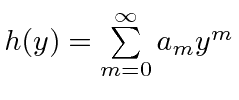and find the recursion relation for the coefficients.
b)
Use the requirement that this polynomial series must terminate to find the allowed energies in terms of.
c)
Findfor the ground state and second excited state.
6. A beam of particles of energycoming fromis incident upon a potential step in one dimension. That is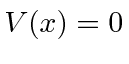forandforwhereis a positive real number.
a)
Find the solution to the Schrödinger equation for this problem.
b)
Determine the coefficients needed to satisfy the boundary conditions.
c)
Calculate the probability for a particle in the beam to be reflected by the potential step and the probability to be transmitted.
7. * A particle is in the ground state (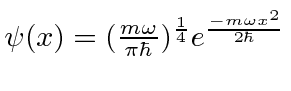.) of a harmonic oscillator potential. Suddenly the potential is removed without affecting the particle's state. Find the probability distributionfor the particle's momentum after the potential has been removed.
8. * A particle is in the third excited state (n=3) of the one dimensional harmonic oscillator potential.
a)
Calculate this energy eigenfunction, up to a normalization factor, from the recursion relations given on the front of the exam.
b)
Give, but do not evaluate, the expression for the normalization factor.
c)
Atthe potential is suddenly removed so that the particle is free. Assume that the wave function of the particle is unchanged by removing the potential. Write an expression for the probability that the particle has momentum in the rangefor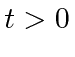. You need not evaluate the integral.
9. * The Schrödinger equation for the one dimensional harmonic oscillator is reduced to the following equation for the polynomial:a)
Assumeand find the recursion relation for the coefficients.
b)
Use the requirement that this polynomial series must terminate to find the allowed energies in terms of.
c)
Findfor the ground state and second excited state.
10. * Find the energy eigenstates (and energy eigenvalues) of a particle of massbound in the 1D potential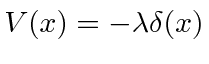.

Jim Branson 2013-04-22